# NCERT Solutions for Class 6 Maths Chapter 7 Fractions in Hindi

## NCERT Solutions for Class 6 Maths Chapter 7 Fractions in Hindi PDF Download

Download the Class 6 Maths NCERT Solutions in Hindi medium and English medium as well offered by the leading e-learning platform Vedantu. If you are a student of Class 6, you have reached the right platform. The NCERT Solutions for Class 6 Maths in Hindi provided by us are designed in a simple, straightforward language, which are easy to memorise. You will also be able to download the PDF file for NCERT Solutions for Class 6 Maths in English and Hindi from our website at absolutely free of cost. Subjects like Science, Maths, English,Hindi will become easy to study if you have access to NCERT Solution for Class 6 Science , Maths solutions and solutions of other subjects.

NCERT, which stands for The National Council of Educational Research and Training, is responsible for designing and publishing textbooks for all the classes and subjects. NCERT textbooks covered all the topics and are applicable to the Central Board of Secondary Education (CBSE) and various state boards.

We, at Vedantu, offer free NCERT Solutions in English medium and Hindi medium for all the classes as well. Created by subject matter experts, these NCERT Solutions in Hindi are very helpful to the students of all classes.

Do you need help with your Homework? Are you preparing for Exams?
Study without Internet (Offline)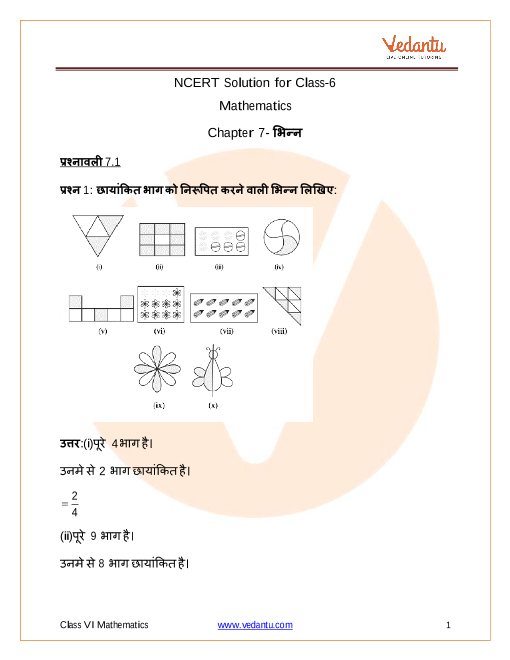## NCERT Solutions for Class 6 Maths Chapter 7 Fractions in Hindi

### प्रश्नावली 7.1

1. छायांकित भाग को निरूपित करने वाली भिन्न लिखिए: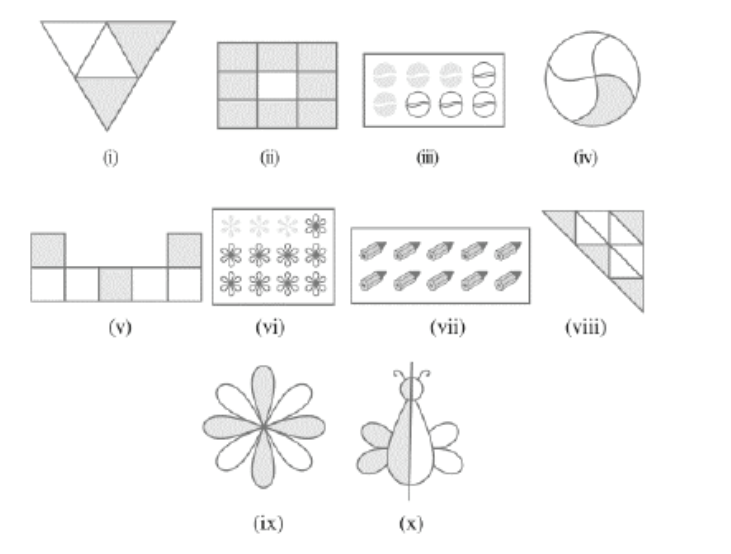उत्तर:

1. पूरे 4भाग है।

उनमे से $2$ भाग छायांकित है।

तब, छायांकित भाग को निरूपित करने वाली भिन्न$=\frac{2}{4}$

1.  पूरे $9$ भाग है।

उनमे से $8$ भाग छायांकित है।

तब, छायांकित भाग को निरूपित करने वाली भिन्न$=\frac{8}{9}$

1. पूरे $8$ भाग है।

उनमे से $4$ भाग छायांकित है।

तब, छायांकित भाग को निरूपित करने वाली भिन्न$=\frac{4}{8}$

1. पूरे $4$ भाग है।

उनमे से $1$ भाग छायांकित है।

तब, छायांकित भाग को निरूपित करने वाली भिन्न$=\frac{1}{4}$

1. पूरे $7$ भाग है।

उनमे से $3$ भाग छायांकित है।

तब, छायांकित भाग को निरूपित करने वाली भिन्न$=\frac{3}{7}$

1.  पूरे $12$ भाग है।

उनमे से $3$ भाग छायांकित है।

तब, छायांकित भाग को निरूपित करने वाली भिन्न$=\frac{3}{12}$

1.  पूरे $10$ भाग है।

उनमे से $10$ भाग छायांकित है।

तब, छायांकित भाग को निरूपित करने वाली भिन्न$=\frac{10}{10}$

1. पूरे $9$ भाग है।

उनमे से $4$ भाग छायांकित है।

तब, छायांकित भाग को निरूपित करने वाली भिन्न$=\frac{4}{9}$

1. पूरे $8$ भाग है।

उनमे से $4$ भाग छायांकित है।

तब, छायांकित भाग को निरूपित करने वाली भिन्न$=\frac{4}{8}$

1. पूरे $2$ भाग है।

उनमे से $1$ भाग छायांकित है।

तब, छायांकित भाग को निरूपित करने वाली भिन्न$=\frac{1}{2}$

2. दी हुई भिन्न के अनुसार, भागों को छायांकित कीजिए: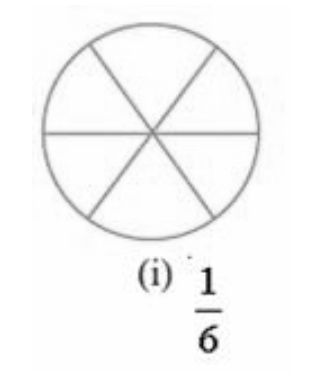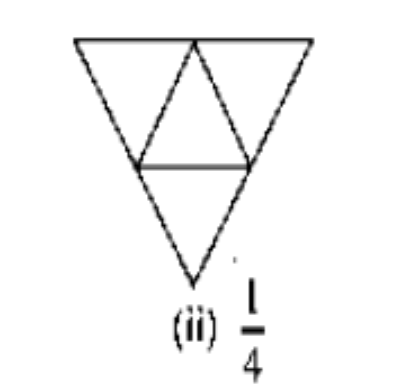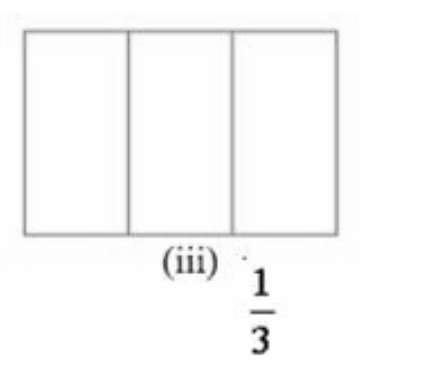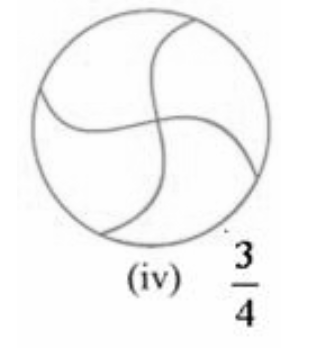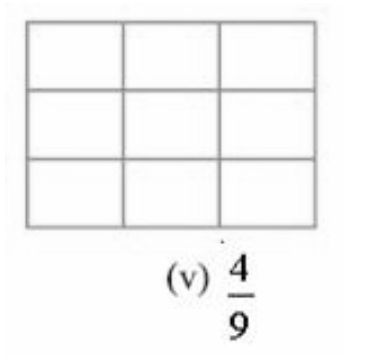उत्तर: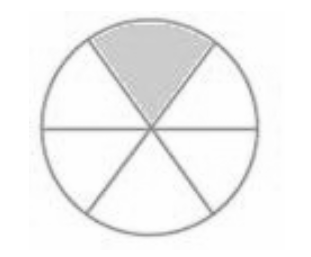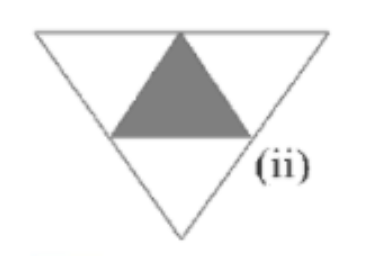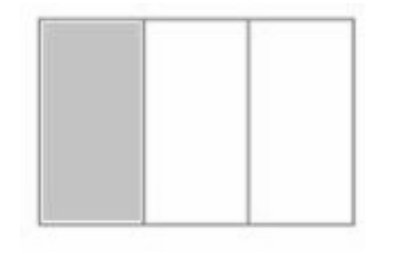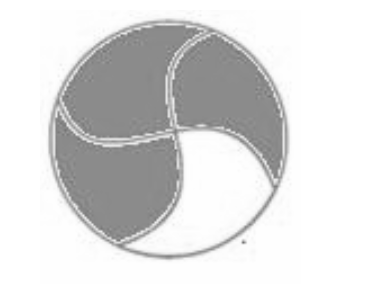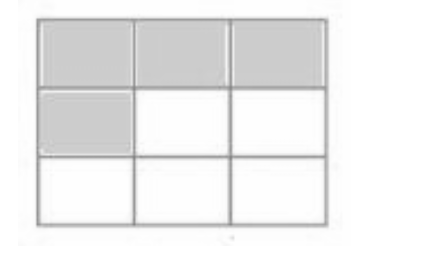3. निम्न में, यदि कोई गलती है, तो पहचानिए: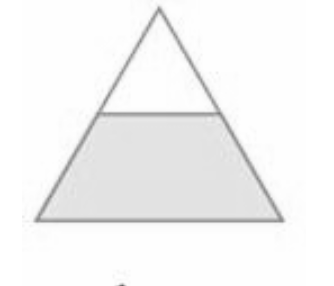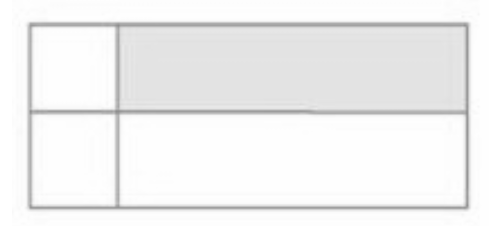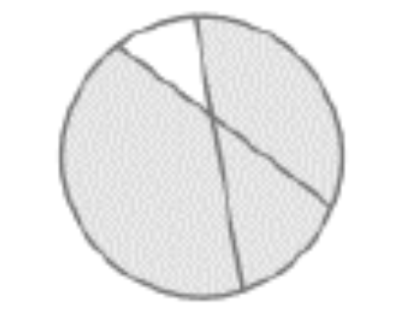उत्तर: तीनों रेखाचित्र को बराबर नही बांटा गया है।

4. $\mathbf{8}$घंटे एक दिन की कौन-सी भिन्न है?

उत्तर: 1 दिन में $24$ घंटे होते हैं।
इसलिए $\mathbf{8}$ घंटे $=\frac{8}{24}=\frac{1}{3}$

5. $\mathbf{40}$ मिनट एक घंटे की कौन-सी भिन्न है?

उत्तर: $1$ घंटे में $60$ मिनट होते हैं।

इसलिए $40$मिनट $=\frac{40}{60}=\frac{2}{3}$

6. आर्या, अभिमन्यु और विवेक एक साथ, बाँटकर खाना खाते हैं। आर्या दो सैंडविच लेकर आता है- एक सब्जी वाला और दूसरा जैम (Jam) वाला। अन्य दो लड़के अपना खाना लाना भूल गए। आर्या अपने सैंडविचों को उन दोनों के साथ बाँटकर खाने को तैयार हो जाता है, ताकि प्रत्येक व्यक्ति को प्रत्येक सैंडविच में से बराबर भाग मिले।

1. आर्या अपनी सैंडविचों को किस प्रकार बाँटे कि प्रत्येक को बराबर भाग मिले?

उत्तर: (a)दोनों सैंडविच को तीन बराबर भागों में बाँट लेंगे।

1. प्रत्येक लड़के को एक सैंडविच का कौन-सा भाग मिलेगा?

उत्तर: एक सैंडविच का $\frac{1}{3}$ भाग मिलेगा।

7. कंचन ड्रेसों (Dresses) को रंगती है। उसे $\mathbf{30}$ड्रेस रंगनी थी। उसने अब तक $\mathbf{20}$ ड्रेस रंग ली हैं। उसने ड्रेसों की कितनी भिन्न रंग ली हैं?

उत्तर: रंगने को मिले ड्रेस$=30$

अभी तक रंगे ड्रेस $=20$

रंगे हुए ड्रेस का भिन्न $=$रंगे ड्रेस /कुल रंगने को मिले ड्रेस

$=\frac{20}{30}$

$= \frac{2}{3}$

8. $\mathbf{2}$ से $\mathbf{12}$ तक की प्राकृत संख्याएँ लिखिए। अभाज्य संख्याएँ इनकी कौन-सी भिन्न हैं?

उत्तर: $\mathbf{2}$ से $\mathbf{12}$ तक की प्राकृत संख्याएँ $=2,\text{ }3,\text{ }4,\text{ }5,\text{ }6,\text{ }7,\text{ }8,\text{ }9,\text{ }10,\text{ }11,\text{ }12$

अभाज्य संख्याएँ $=2,\text{ }3,\text{ }5,\text{ }7,\text{ }11$

कुल संख्याएँ $=11$

अभाज्य संख्याएँ $=5$

अभाज्य संख्याओं का भिन्न $=\frac{5}{11}$

9. $\mathbf{102}$से $\mathbf{113}$ तक की प्राकृत संख्याएँ लिखिए। अभाज्य संख्याएँ इनकी कौन-सी भिन्न हैं?

उत्तर:

$\mathbf{102}$से $\mathbf{113}$ तक की प्राकृत संख्याएँ

$= 102, 103, 104, 105, 106, 107, 108, 109,110,111,112,113$

प्राकृत कुल संख्या $=12$

अभाज्य संख्याएँ $=103,\text{ }107,\text{ }109,\text{ }113$

अभाज्य  कुल संख्या $=4$

अभाज्य संख्याओं का भिन्न $=\frac{4}{12}=\frac{1}{3}$

10. इन वृतों की कौन-सी भिन्नों में $\mathbf{X}$ है?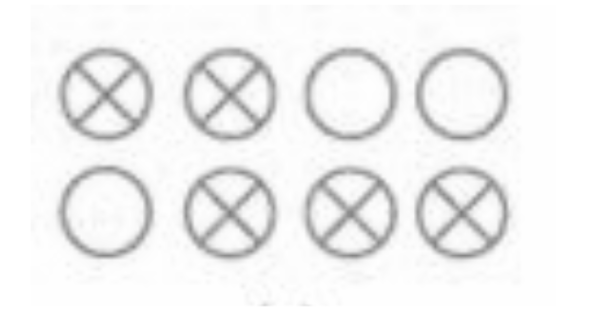उत्तर:पूरे वृत $=8$

वृतों मे $x$ =4

$x$ वृतों के भिन्न = $x$ वृत्तो / पूरे वृत्त

$=\frac{4}{8}$

11. क्रिस्तिन अपने जन्म दिन पर एक सीडी प्लेयर (CD player) प्राप्त करती है। वह तब से सीडी इकट्ठी करना प्रारंभ कर देती है। वह $\mathbf{3}$ सीडी खरीदती है और $\mathbf{5}$सीडी उपहार के रूप में प्राप्त करती है। उसके द्वारा खरीदी गई सीडी की संख्या, कुल सीडी की संख्या की कौन-सी भिन्न है?

उत्तर:

खरीदी हुई सीडी$=3$

उपहार मे मिली सीडी$=5$

सीडी की कुल संख्या = 3 + 5 = 8

क्रिस्तिन द्वारा खरीदी गई सीडी की कुल संख्या = 3

खरीदी गई सीडी का भिन्न $=$खरीदी गई सीडी/कुल सीडी

$=\frac{3}{8}$

### प्रश्नावली 7.2

1. संख्या रेखाएँ खींचिए और उस पर निम्नलिखित भिन्नों को बिंदु के रूप में दर्शाइए:

1. $\frac{1}{2}, \frac{1}{4}, \frac{3}{4}, \frac{4}{4}$

2. $\frac{1}{8}, \frac{2}{8}, \frac{3}{8}, \frac{7}{8}$

3. $\frac{2}{5}, \frac{3}{5}, \frac{8}{5}, \frac{4}{5}$

उत्तर: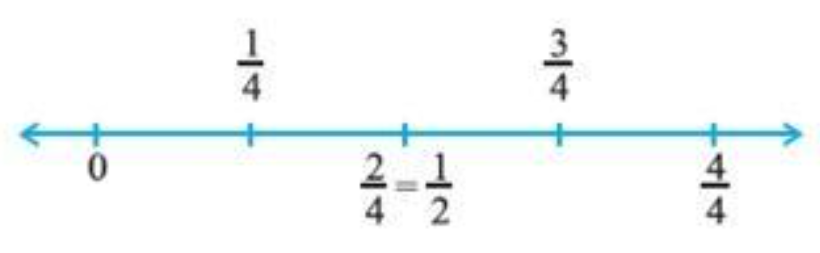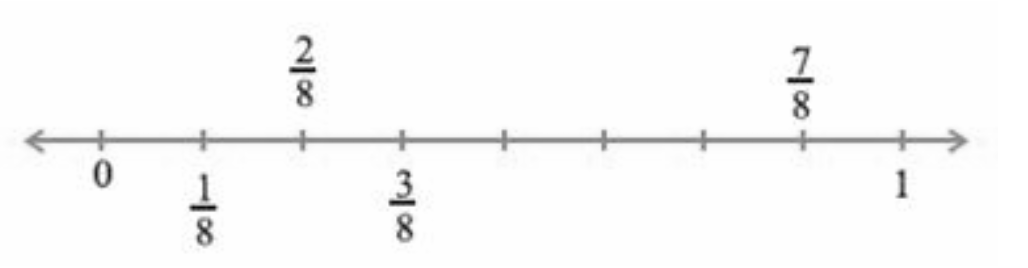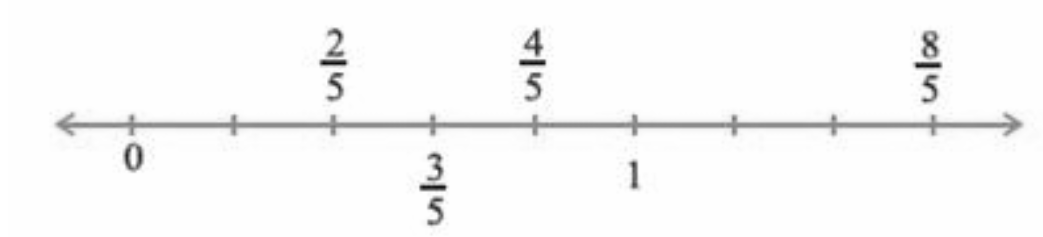2.  निम्नलिखित को मिश्रित भिन्न के रूप में व्यक्त कीजिए:
$\left( a \right)~\frac{20}{3}~\left( b \right)~\frac{11}{5}~\left( c \right)\frac{17}{7}~~\left( d \right)~\frac{28}{5}~\left( e \right)~\frac{19}{6}~\left( f \right)~\frac{35}{9}$

उत्तर: $\left( a \right)~6\frac{2}{3}~\left( b \right)~2\frac{1}{5}~\left( c \right)2\frac{3}{7}~~\left( d \right)~5\frac{3}{5}~\left( e \right)~3\frac{1}{6}~\left( f \right)3~\frac{8}{9}$

3. निम्नलिखित को विषम भिन्न के रूप में व्यक्त कीजिए: $\left( a \right)~7\frac{3}{4}~\left( b \right)~5\frac{6}{7}~\left( c \right)2\frac{5}{6}~~\left( d \right)~10\frac{3}{5}~\left( e \right)~9\frac{3}{7}~\left( f \right)8\frac{4}{9}$

उत्तर:

1. $7 \frac{3}{4} = \frac{(7 \times 4)+3}{4}= \frac{31}{4}$

2. $5\frac{6}{7}=\frac{(7 \times 5)+6}{7}= \frac{41}{7}$

3. $2\frac{5}{6} = \frac{(6\times 2)+5}{6}=\frac{17}{6}$

4. $10 \frac{3}{5} =\frac{(10\times 5)+3}{5}=\frac{53}{5}$

5. $9\frac{3}{7}=\frac{(7 \times 9)+3}{7}=\frac{67}{7}$

6. $8\frac{4}{9}=\frac{(9\times 8)+4}{9}=\frac{76}{9}$

### प्रश्नावली 7.3

1. प्रत्येक चित्र में छायांकित भागों के लिए भिन्न लिखिए। क्या ये सभी भिन्न तुल्य है?
a.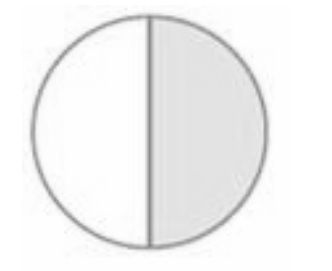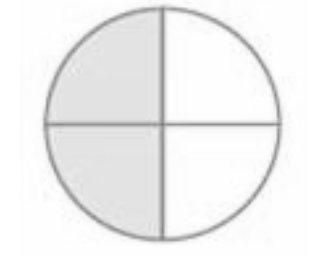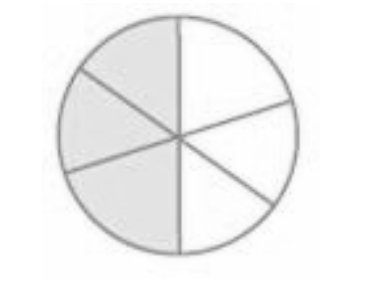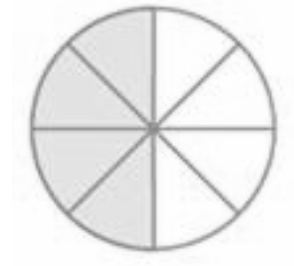उत्तर:  $(a)\left( \frac{1}{2},\frac{2}{4},\frac{3}{6},\frac{4}{8} \right)$हाँ, यह सभी तुल्य भिन्न है।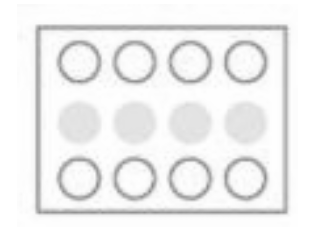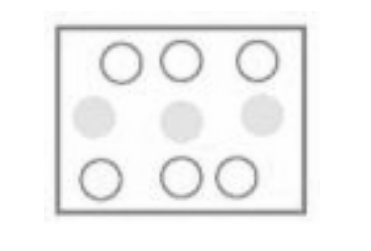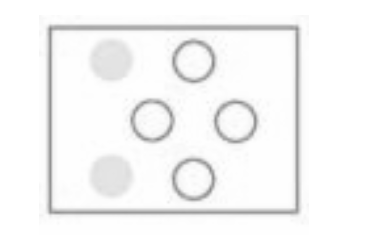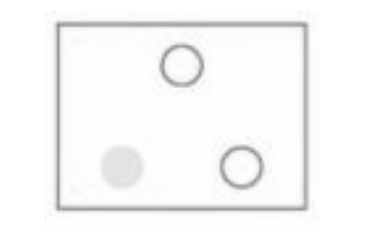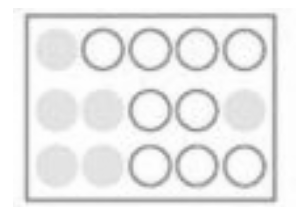b. उत्तर: $(b)\left( \frac{4}{12},\frac{3}{9},\frac{2}{6},\frac{1}{3},\frac{6}{15} \right)$ नहीं , ये सभी तुल्य भिन्न नही है क्योकि ये आस पास मे बराबर नही है।

2.छायांकित भागो के लिए भिन्नों को लिखिए और प्रत्येक पंक्ति में से तुल्य भिन्नों को चुनिए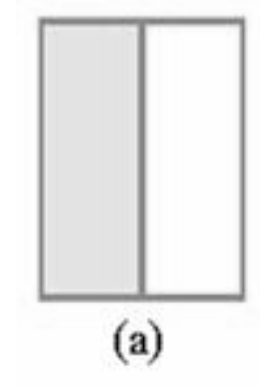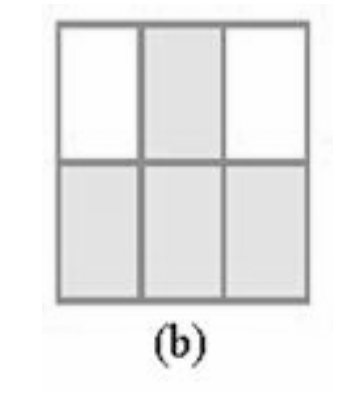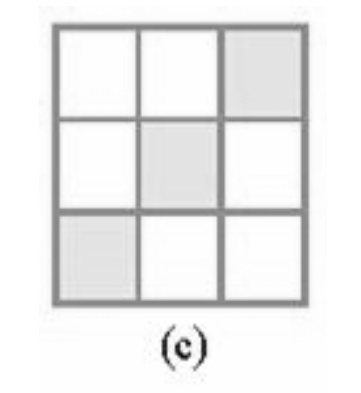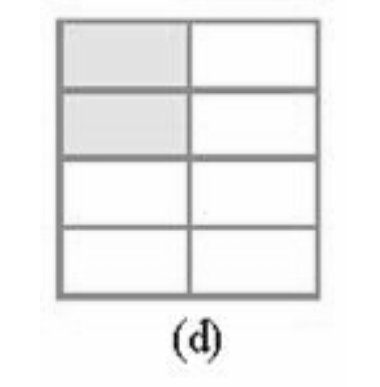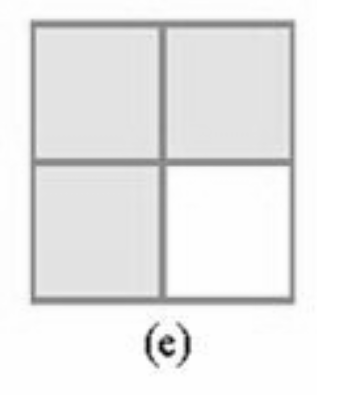उत्तर: $(a)\frac{1}{2},(b)\frac{4}{6},(c)\frac{3}{9},(d)\frac{2}{8},(e)\frac{3}{4}$

उत्तर:$(i)\frac{6}{18},(ii)\frac{4}{8},(iii)\frac{4}{16},(iv)\frac{4}{12},(v)\frac{4}{16}$

तुल्य भिन्न $:\text{ }(a)\text{ }ii\text{ }\left( b \right)\text{ }i\text{ }\left( c \right)\text{ }iv\text{ }\left( d \right)\text{ }iii\text{ }\left( e \right)\text{ }v$

3. निम्न में से प्रत्येक में $\square$ को सही संख्या से प्रतिस्थापित कीजिए:
$(a)\frac{2}{7}=\frac{8}{}(b)\frac{5}{10}=\frac{10}{}(c)\frac{3}{5}=\frac{}{20}(d)\frac{45}{60}=\frac{15}{}(e)\frac{18}{24}=\frac{}{4}$

उत्तर: $\left( a \right)\frac{2}{7}=\frac{2\cdot 4}{7\cdot 4}=\frac{8}{\overline{\underline{\left| 28 \right|}}}$

$\left( b \right)\frac{5}{8}=\frac{5\cdot 2}{8\cdot 2}=\frac{10}{\overline{\underline{\left| 16 \right|}}}$

$\left( c \right)\frac{3}{5}=\frac{3\times 4}{5\times 4}=\frac{\overline{\underline{\left| 12 \right|}}}{20}$

$\left( d \right)\frac{45}{60}=\frac{45\div 3}{60\div 3}=\frac{15}{\overline{\underline{\left| 20 \right|}}}$

$\left( e \right)\frac{18}{24}=\frac{18\div 6}{24\div 6}=\frac{\overline{\underline{\left| 3 \right|}}}{4}$

4. $\frac{3}{5}$के तुल्य वह भिन्न ज्ञात कीजिए जिसका
(a) हर $20$ है  (b) अंश $9$ है

(c) हर $30$ है   (d) अंश $27$ है

उत्तर:

1. $\frac{3}{5}=\frac{3\times 4}{5\times 4}=\frac{12}{20}$

2. $\frac{3}{5}=\frac{3\times 3}{5\times 3}=\frac{9}{15}$

3. $\frac{3}{5}=\frac{3\times 6}{5\times 6}=\frac{18}{30}$

4. $\frac{3}{5}=\frac{3\times 9}{5\times 9}=\frac{27}{45}$

5. $\frac{36}{48}$ के तुल्य वह भिन्न ज्ञात कीजिए जिसका
(a) अंश $9$ है (b) हर $4$ है

उत्तर:

1. $\frac{36}{48}=\frac{36\div 4}{48\div 4}=\frac{9}{12}$

2. $\frac{36}{48}=\frac{36\div 12}{48\div 12}=\frac{3}{4}$

6. जाँच कीजिए कि निम्न भिन्न तुल्य है या नहीं:
$(a)\frac{5}{9},\frac{30}{34}$

उत्तर: दोनों का हर बराबर करने पर:
$\frac{5\times 6}{9\times 6}=\frac{30}{54}$

$\frac{5}{9},\frac{30}{54}$

$(b)\frac{3}{10},\frac{12}{54}$

उत्तर: दोनों का हर बराबर करने पर:
$\frac{3\times 5}{10\times 5}=\frac{15}{50}$
$\frac{3}{10},\frac{12}{54}$तुल्य नहीं हैं।

$(c)\frac{7}{13},\frac{5}{11}$

उत्तर: दोनों का हर बराबर करने पर:

$\frac{7\times 11}{13\times 11}=\frac{77}{143}$

$\frac{5\times 13}{11\times 13}=\frac{55}{143}$

$\frac{7}{13},\frac{5}{11}$तुल्य नहीं हैं।

7. निम्नलिखित भिन्नों को सरलतम रूप में बदलिए:

1. $\frac{48}{60}$

2. $\frac{150}{60}$

3. $\frac{84}{98}$

4. $\frac{12}{52}$

5. $\frac{7}{28}$

उत्तर:

1. $\frac{48}{60}=\frac{4}{5}$

2. $\frac{150}{60}=\frac{5}{2}$

3. $\frac{84}{98}=\frac{6}{7}$

4. $\frac{12}{52}=\frac{7}{13}$

5. $\frac{7}{28}=\frac{1}{4}$

8. रमेश के पास 20 पेंसिल थी। शीलू के पास $\mathbf{50}$ पेंसिल जमाल के पास 80 पेंसिल थीं। $\mathbf{4}$ महीने के बाद रमेश ने $\mathbf{10}$ पेंसिल तथा शीलू ने $\mathbf{25}$ पेंसिल प्रयोग कर ली और जमाल ने $\mathbf{40}$पेंसिल प्रयोग कर ली। प्रत्येक ने अपनी पैंसिलों की कौन-सी भिन्न प्रयोग कर ली? जाँच कीजिए कि प्रत्येक ने अपनी पैंसिलों की समान भिन्न प्रयोग की है।

उत्तर:रमेश के लिए :

कुल पेंसिल$=20$

प्रयोग की गई पेंसिल$=10$

रमेश द्वारा इस्तेमाल हुई पेंसिल भिन्न मे  $=\frac{10}{20}=\frac{1}{2}$

शीलू के लिए

कुल पेंसिल$=50$

प्रयोग की गई पेंसिल $=25$

शीलू द्वारा इस्तेमाल हुई पेंसिल  भिन्न मे $=\frac{25}{50}=\frac{1}{2}$

जमाल के लिए ;

कुल पेंसिल $=80$

प्रयोग की गई पेंसिल$=40$

जमाल द्वारा इस्तेमाल हुई पेंसिल भिन्न मे $=\frac{40}{80}=\frac{1}{2}$

यह स्पष्ट है प्रत्येक ने अपनी पैंसिलों की $\frac{1}{2}$ भिन्न प्रयोग कर ली, हाँ,प्रत्येक ने अपनी पैंसिलों की समान भिन्न प्रयोग किया है।

9. तुल्य भिन्नों का मिलान कीजिए और प्रत्येक के लिए दो भिन्न और लिखिए :

 (i) $\frac{250}{400}$ (a) $\frac{2}{3}$ (ii) $\frac{180}{200}$ (b) $\frac{2}{5}$ (iii) $\frac{660}{990}$ (c) $\frac{1}{2}$ (iv) $\frac{180}{360}$ (d) $\frac{5}{8}$ (v) $\frac{220}{550}$ (e) $\frac{9}{10}$

उत्तर:

(i) $\frac{250}{400}$$=\frac{5}{8},\frac{10}{16},\frac{15}{24}$     (d) $\frac{5}{8}$

(ii) $\frac{180}{200}=\frac{9}{10},\frac{18}{20},\frac{27}{30}$                        (e ) $\frac{9}{10}$

(iii) $\frac{660}{990}=\frac{2}{3},\frac{4}{6},\frac{6}{9}$                     (a) $\frac{2}{3}$

(iv) $\frac{180}{360}=\frac{1}{2},\frac{2}{4},\frac{3}{6}$                                (c) $\frac{1}{2}$

(v) $\frac{220}{550}=\frac{2}{5},\frac{4}{10},\frac{6}{15}$                                  ( b) $\frac{2}{5}$

### प्रश्नावली 7.4

1. प्रत्येक चित्र के लिए भिन्नों को लिखिए। भिन्नों के बीच में सही चिन्ह $<,\text{ }=,\text{ }>$का प्रयोग करते हुए, इन्हें आरोही और अवरोही क्रम में व्यवस्थित कीजिए: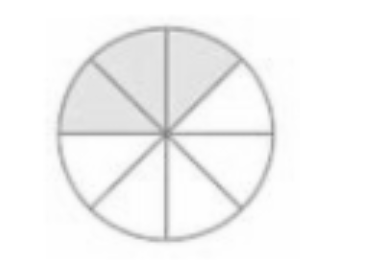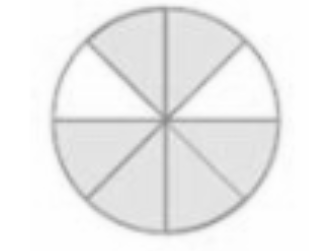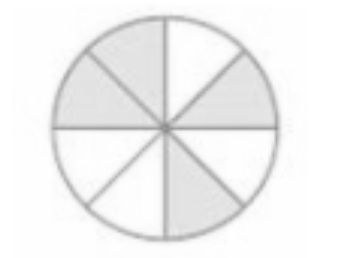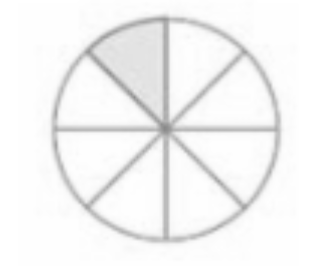उत्तर: आरोही क्रम $=\frac{1}{8}~<\frac{3}{8}~<~\frac{4}{8}~<~\frac{6}{8}$

अवरोही क्रम $=\frac{6}{8}~>\frac{4}{8}~>~\frac{3}{8}~>~\frac{1}{8}$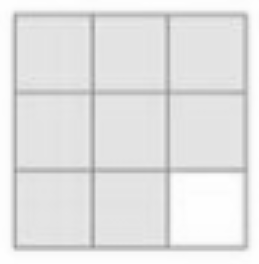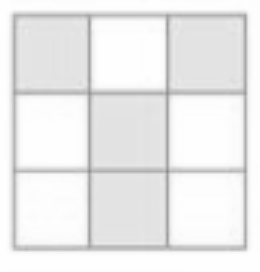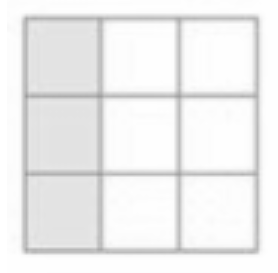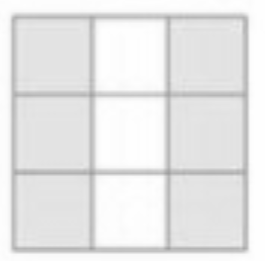उत्तर: आरोही क्रम $=\frac{3}{9}~<\frac{4}{9}~<~\frac{6}{9}~<~\frac{8}{9}$

अवरोही क्रम $=\frac{8}{9}~>\frac{6}{9}~>~\frac{4}{9}~>~\frac{3}{9}$

1.  $\frac{2}{6}~,\frac{4}{6}~,\frac{8}{6}$और $\frac{6}{6}$ को संख्या रेखा पर दर्शाइए।

उत्तर: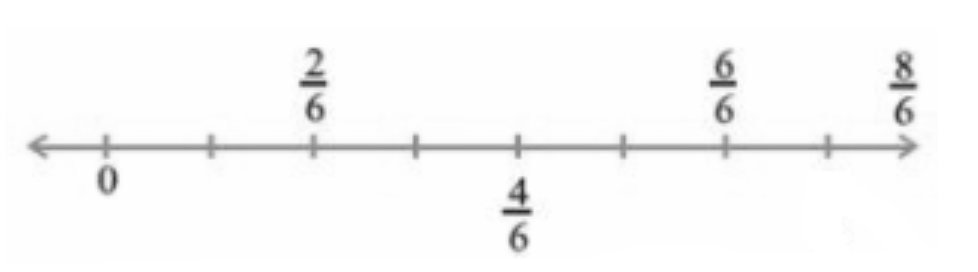1. दी हुई भिन्न के बीच में उचित चिन्ह ‘$<$’ या ‘$>$’ भरिए:

$\frac{5}{6}\square\frac{2}{6}~,\frac{3}{6}\square0,~\frac{1}{6}\square\frac{6}{6},\frac{8}{6}\square\frac{5}{6}$

उत्तर: $\frac{5}{6}~>\frac{2}{6}~,\frac{3}{3}>0,~\frac{1}{6}>\frac{6}{6},\frac{8}{6}>\frac{5}{6}$

यदि भिन्नों के हर बराबर हों तो बड़े अंश वाला भिन्न बड़ा होता है।

2. भिन्नों की तुलना कीजिए और उचित चिन्ह लगाइए:

1. $\frac{3}{6} \square \frac{5}{6}$

2. $\frac{1}{7}\square \frac{1}{4}$

3. $\frac{4}{5}\square \frac{5}{5}$

4. $\frac{3}{5}\square\frac{3}{7}$

उत्तर:

1. $\frac{3}{6} < \frac{5}{6}$

2. $\frac{1}{7} < \frac{1}{4}$

3. $\frac{4}{5} < \frac{5}{5}$

4. $\frac{3}{5} > \frac{3}{7}$

यदि भिन्नों के हर बराबर हों तो बड़े अंश वाला भिन्न बड़ा होता है। यदि भिन्नों के अंश बराबर हों तो बड़े हर वाला भिन्न छोटा होता है।

3. ऐसे ही और पाँच युग्म लीजिए और उचित चिन्ह$\left( <,> \right)$ लगाइए।

उत्तर: $\frac{5}{6}>\frac{2}{6}~,\frac{3}{3}>0,~\frac{1}{6}<\frac{6}{6},\frac{8}{6}>\frac{5}{6},\frac{1}{7}<\frac{1}{4}$

4. निम्न आकृतियों को देखिए और भिन्नों के बीच में उचित चिन्ह $>,\text{ }=$, या $<$  लिखिए: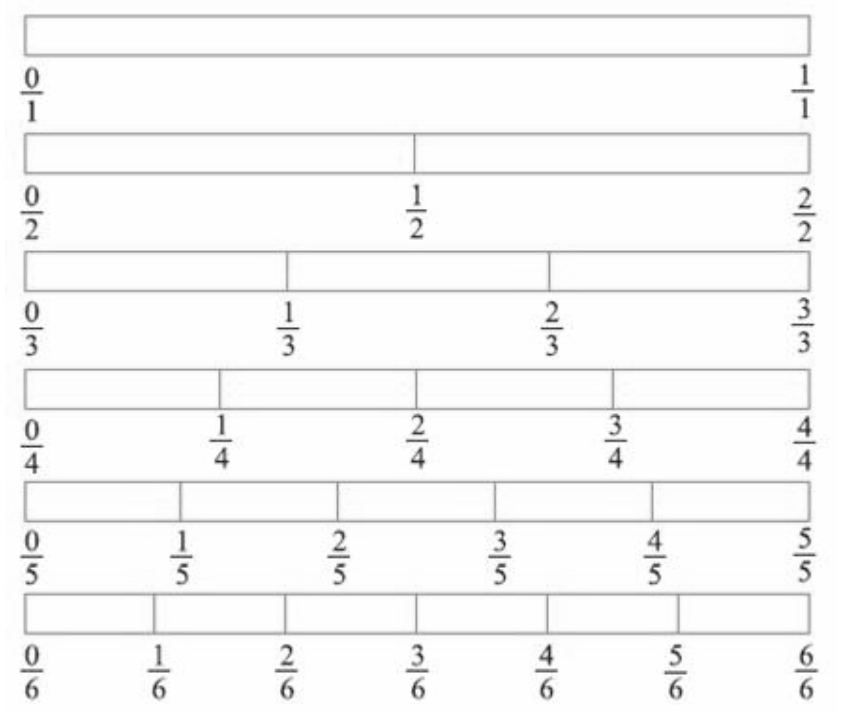$(a)\frac{1}{6} \square \frac{1}{3}$         $(b)\frac{3}{4} \square \frac{2}{6}$              $(c)\frac{2}{3} \square \frac{2}{4}$

$(d)\frac{6}{6} \square \frac{3}{3}$           $(e)\frac{5}{6} \square \frac{5}{5}$

उत्तर:

1. $\frac{1}{6} < \frac{1}{3}$

2. $\frac{3}{4} > \frac{2}{6}$

3. $\frac{2}{3} > \frac{2}{4}$

4. $\frac{6}{6}=\frac{3}{3}$

5. $\frac{5}{6} < \frac{5}{5}$

ऐसे ही पाँच और प्रश्न बनाइए और अपने मित्रों के साथ उन्हें हल कीजिए।

$\left( i \right)\frac{1}{2}=\frac{4}{8},\left( ii \right)\frac{2}{3}>\frac{4}{6},\left( iii \right)\frac{5}{6}<\frac{2}{2},\left( iv \right)\frac{1}{1}=\frac{2}{2}$

5. देखें कितनी जल्दी आप करते हैं? उचित चिन्ह भरिए $(<,\text{ }=,\text{ }>):$

$(a)\frac{1}{2}\square\frac{1}{5}$           $(b)\frac{2}{4}\square\frac{3}{6}$            $(c)\frac{3}{5}\square\frac{2}{3}$

$(d)\frac{3}{4}\square \frac{2}{8}$           $(e)\frac{3}{5}\square\frac{6}{5}$             $(f)\frac{7}{9}\square\frac{3}{9}$

$(g)\frac{1}{4}\square\frac{2}{8}$            $(h)\frac{6}{10}\square\frac{4}{5}$          $(i)\frac{3}{4}\square\frac{7}{8}$

$(j)\frac{6}{10}\square\frac{3}{5}$            $(k)\frac{5}{7}\square\frac{15}{21}$

उत्तर:

1. $\frac{1}{2} > \frac{1}{5}$

2. $\frac{2}{4}=\frac{3}{6}$

3. $\frac{3}{5} < \frac{2}{3}$

4. $\frac{3}{4} > \frac{2}{8}$

5. $\frac{3}{5} < \frac{6}{5}$

6. $\frac{7}{9} > \frac{3}{9}$

7. $\frac{1}{4} = \frac{2}{8}$

8. $\frac{6}{10} < \frac{4}{5}$

9. $\frac{3}{4} < \frac{7}{8}$

10. $\frac{6}{10} < \frac{3}{5}$

11. $\frac{5}{7} = \frac{15}{21}$

6. निम्नलिखित भिन्न तीन अलग-अलग संख्याएँ निरूपित करती है इन्हें सरलतम रूप में बदलकर उन तीन तुल्य भिन्नों के समूहों में लिखिए:

$(a)\frac{2}{12}$       $(b)\frac{3}{15}$           $(c)\frac{8}{50}$

$(d)\frac{16}{100}$         $(e)\frac{10}{60}$          $(f)\frac{15}{75}$

$(g)\frac{12}{60}$           $(h)\frac{16}{96}$           $(i)\frac{12}{75}$

$(j)\frac{12}{72}$            $(k)\frac{3}{18}$            $(l)\frac{4}{25}$

उत्तर:

1. $\frac{2}{12}=\frac{1}{6}$

2. $\frac{3}{15}=\frac{1}{5}$

3. $\frac{8}{50}=\frac{4}{25}$

4. $\frac{16}{100}=\frac{4}{25}$

5. $\frac{10}{60}=\frac{1}{6}$

6. $\frac{15}{75}=\frac{1}{5}$

7. $\frac{12}{60}=\frac{1}{5}$

8. $\frac{16}{96}=\frac{1}{6}$

9. $\frac{12}{75}=\frac{4}{25}$

10. $\frac{12}{72}=\frac{1}{6}$

11. $\frac{3}{18}=\frac{1}{6}$

12. $\frac{4}{25}=\frac{4}{25}$

7. निम्नलिखित के उत्तर दीजिए। लिखिए और दर्शाइए कि आपने इन्हें कैसे हल किया है?

1. क्या $\frac{5}{9},\frac{4}{5}$के बराबर है?

उत्तर: दोनों के हर बराबर करने के लिए,

$\frac{5}{9}\times \frac{5}{5}=\frac{25}{45}$

$\frac{4}{5}\times \frac{9}{9}=\frac{36}{45}$

$\frac{25}{45}\ne \frac{36}{45}$

इसलिए $\frac{5}{9}\ne \frac{4}{5}$

1. क्या $\frac{9}{16},\frac{5}{9}$के बराबर है?

उत्तर: दोनों के हर बराबर करने के लिए ,

$\frac{9}{16}\times \frac{9}{9}=\frac{81}{144}$

$\frac{5}{9}\times \frac{16}{16}=\frac{80}{144}$

इसलिए $\frac{9}{16}\ne \frac{5}{9}$

1. क्या $\frac{4}{5},\frac{16}{20}$के बराबर है?

उत्तर: दोनों के हर बराबर करने पर

$\frac{4}{5}\times \frac{4}{4}=\frac{16}{20}$

इसलिए $\frac{4}{5}=\frac{16}{20}$

1. क्या $\frac{1}{15},\frac{4}{30}$के बराबर है?

उत्तर: दोनों के हर बराबर करने पर

$\frac{1}{15}\times \frac{4}{30}=\frac{2}{30}$

इसलिए $\frac{1}{15}\ne \frac{4}{30}$

8. इला $\mathbf{100}$ पृष्ठों वाली एक पुस्तक के $\mathbf{25}$ पृष्ठ पढ़ती है। ललिता इसी पुस्तक का $\frac{1}{2}$ भाग पढ़ती है। किसने कम पढ़ा?

उत्तर: इला $100$ पृष्ठो वाली एक पुस्तकके $25$ पृष्ठ पढ़ती है।

इसीलिए पढे गए प्रश्नो की भिन्न =$\frac{25}{100}=\frac{1}{4}$

ललिता द्वारा पढ़ा गया भाग $=\frac{1}{2}=50$ पेज

क्योकि, $\frac{1}{4}<\frac{1}{2}$

अतः इला ने कम पढ़ा है।

9. रफीक ने एक घंटे के $\frac{3}{6}$ भाग तक व्यायाम किया, जबकि रोहित ने एक घंटे के $\frac{3}{4}$ भाग तक व्यायाम किया। किसने लंबे समय तक व्यायाम किया?

उत्तर: $1$घंटा = $60$ मिनट

रफीक ने एक घंटे के $\frac{3}{6}$ भाग तक व्यायाम किया

$=\frac{3}{6}\times 60=30$ मिनट

रोहित ने एक घंटे के $\frac{3}{4}$ भाग तक व्यायाम किया

$=\frac{3}{4}\times 60=45$ मिनट

अतः $\frac{3}{6}<\frac{3}{4}$

इसीलिए रोहित ने अधिक समय तक कसरत किया।

10. $\mathbf{25}$ विद्यार्थियों की एक कक्षा $\mathbf{A}$ में $\mathbf{20}$ विद्यार्थी $\mathbf{60}%$या अधिक अंक लेकर पास हुए और $\mathbf{30}$ विद्यार्थियों की एक कक्षा $\mathbf{B}$ में $\mathbf{24}$विद्यार्थी $\mathbf{60}%$या अधिक अंक लेकर पास हुए। किस कक्षा में विद्यार्थियों का अधिक भाग $\mathbf{60}%$या अधिक अंक लेकर पास हुआ?

उत्तर: कक्षा $A$ में $60%$ या अधिक अंक वाले विद्यार्थियों का भाग

$=\frac{20}{25}=\frac{4}{5}$

कक्षा $B$ में $60%$ या अधिक अंक वाले विद्यार्थियों का भाग

$=\frac{24}{30}=\frac{4}{5}$

दोनों ही कक्षाओं में 60% या अधिक अंक वाले विद्यार्थियों का भाग बराबर है।

### प्रश्नावली 7.5

1. निम्न भिन्नों को योग या घटाने के उचित रूप में लिखिए: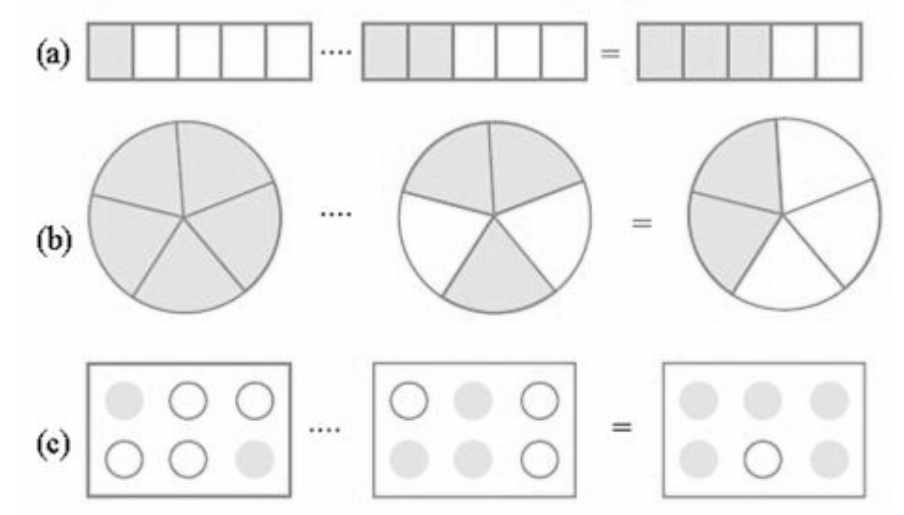उत्तर:

1. $\frac{1}{5}+\frac{2}{5}=\frac{3}{5}$

2. $\frac{5}{5}-\frac{3}{5}=\frac{2}{5}$

3. $\frac{2}{6}+\frac{3}{6}=\frac{5}{6}$

2. हल कीजिए:

$a.\frac{1}{18}+\frac{1}{18}$

उत्तर: $\frac{1}{18}+\frac{1}{18}=\frac{1}{9}$

$b.\frac{8}{15}+\frac{8}{15}$

उत्तर: $\frac{8}{15}+\frac{3}{15}=\frac{8+8}{15}=\frac{11}{15}$

$c.\frac{7}{7}-\frac{5}{7}$

उत्तर: $\frac{7}{7}-\frac{5}{7}=\frac{7-5}{7}=\frac{2}{7}$

$d.\frac{1}{22}+\frac{21}{22}$

उत्तर: $\frac{1}{22}+\frac{21}{22}=\frac{1+21}{22}=\frac{22}{22}=1$

$e.\frac{12}{15}-\frac{7}{15}$

उत्तर: $\frac{12}{15}-\frac{7}{15}=\frac{12-7}{15}=\frac{5}{15}=\frac{1}{3}$

$f.\frac{5}{8}+\frac{3}{8}$

उत्तर: $\frac{5}{8}+\frac{3}{8}=\frac{5+3}{8}=\frac{8}{8}=1$

$g.\left( a-\frac{2}{3} \right)$ जहां $a=\frac{3}{3}$

उत्तर: $\frac{3}{3}-\frac{2}{3}=\frac{3-2}{3}=\frac{1}{3}$

$h.\frac{1}{4}+\frac{0}{4}$

उत्तर: $\frac{1}{4}+\frac{0}{4}=\frac{1}{4}$

$i.3-\frac{12}{5}$

उत्तर: $3-\frac{12}{5}=\frac{15-12}{5}=\frac{3}{5}$

3. शुभम ने अपने कमरे की दीवार के $\frac{2}{3}$ भाग को पेंट किया। उसकी बहन माधवी ने उसकी सहायता की और उस दीवार के $\frac{1}{3}$ भाग पर पेंट किया। उन दोनों ने मिलकर कुल कितना पेंट किया?

उत्तर: शुभम द्वारा कमरे मे पैंट किया गया भाग =$\frac{2}{3}$

माधवी द्वारा कमरे मे पैंट किया गया भाग =$\frac{1}{3}$

कुल किया गया पेंट

$\frac{2}{3}+\frac{1}{3}=\frac{3}{3}=1$

अतः दोनों ने मिलकर पूरी दीवार को पेंट किया।

4. रिक्त स्थानों को भरिए:

$(a)\frac{7}{10}-\square=\frac{3}{10}$         $(b) \square-\frac{3}{21}=\frac{5}{21}$                               $(c)\square-\frac{3}{6}=\frac{3}{6}$       $(d) \square+\frac{5}{27}=\frac{12}{27}$

उत्तर: $(a)\frac{4}{10}$

$(b) \frac{8}{21}$

$(c) \frac{6}{6}$

$(d) \frac{7}{27}$

5. जावेद को संतरों की एक टोकरी का $\frac{5}{7}$ भाग मिला। टोकरी में संतरों का कितना भाग शेष रहा?

उत्तर: कुल भाग =1

इसीलिए टोकरी मे शेष गया भाग $=1-\frac{5}{7}$

$\frac{7-5}{7}=\frac{2}{7}$]

अतः टोकरी मे संतरो का भाग $\frac{2}{7}$ शेष रहा ।

### प्रश्नावली 7.6

1.  हल कीजिए:

$(a)\frac{2}{3}+\frac{1}{7}$

उत्तर: $3$ और $7$ का $L.C.M.\text{ }21$है ।

$\frac{2}{3}+\frac{1}{7}=\frac{14+3}{21}=\frac{17}{21}$

$(b)\frac{3}{10}+\frac{7}{15}$

उत्तर:  $10$ और $15$ का $L.C.M.\text{ }30$है,

$\frac{3}{10}+\frac{7}{15}=\frac{9+14}{30}=\frac{23}{30}$

$(c)\frac{4}{9}+\frac{2}{7}$

उत्तर:  $9$ और $7$ का $L.C.M.\text{ }63$है ।

$\frac{4}{9}+\frac{2}{7}=\frac{28+18}{63}=\frac{46}{63}$

$(d)\frac{5}{7}+\frac{1}{3}$

उत्तर: $7$ और $3$ का $L.C.M.\text{ }21$है

$\frac{5}{7}+\frac{1}{3}=\frac{15+7}{21}=\frac{22}{21}$

$(e)\frac{2}{5}+\frac{1}{6}$

उत्तर:  $5$और $6$का $L.C.M.\text{ }30$है ।

$\frac{2}{5}+\frac{1}{6}=\frac{12+5}{30}=\frac{17}{30}$

$(f)\frac{4}{5}+\frac{2}{3}$

उत्तर: $5$और $3$ का $L.C.M.\text{ }15$है ।

$\frac{4}{5}+\frac{2}{3}=\frac{12+10}{15}=\frac{22}{15}$

$(g)\frac{3}{4}-\frac{1}{3}$

उत्तर: $4$ और $3$का $L.C.M.\text{ }12$है ।

$\frac{3}{4}-\frac{1}{3}=\frac{9-4}{12}=\frac{5}{12}$

$(h)\frac{5}{6}-\frac{1}{3}$

उत्तर: $6$ और $3$ का $L.C.M.\text{ }6$है ।

$\frac{5}{6}-\frac{1}{3}=\frac{5-2}{6}=\frac{3}{6}=\frac{1}{2}$

$(i)\frac{2}{3}+\frac{3}{4}+\frac{1}{2}$

उत्तर: $3,\text{ }4$और$2$का $L.C.M.\text{ }12$है ।

$\frac{2}{3}+\frac{3}{4}+\frac{1}{2}=\frac{8+9+6}{12}=\frac{23}{12}$

$(j)\frac{1}{2}+\frac{1}{3}+\frac{1}{6}$

उत्तर: $2,\text{ }3$और $6$ का $L.C.M.\text{ }6$है ।

$\frac{1}{2}+\frac{1}{3}+\frac{1}{6}=\frac{3+2+1}{6}=\frac{6}{6}=1$

$(k)\frac{4}{3}+\frac{11}{3}$

उत्तर: $3$और $3$ का $L.C.M.\text{ }3$है ।

$\frac{4}{3}+\frac{11}{3}=\frac{4+11}{11}=\frac{15}{3}=5$

$(l)\frac{42}{3}+\frac{31}{4}$

उत्तर: $3$ और $4$ का $L.C.M.\text{ }12$है

$\frac{42}{3}+\frac{31}{4}=\frac{56+39}{12}=\frac{95}{12}=7\frac{11}{12}$

$(m)\frac{16}{5}-\frac{7}{5}$

उत्तर: $5$ और $5$ का $L.C.M.\text{ }5$है ।

$\frac{16}{5}-\frac{7}{5}=\frac{16-7}{5}=\frac{9}{5}$

$(n)\frac{4}{3}-\frac{1}{2}$

उत्तर: $3$और $2$ का $L.C.M.\text{ }6$है ।

$\frac{4}{3}-\frac{1}{2}=\frac{8-3}{6}=\frac{5}{6}$

2. सरिता ने $\frac{2}{5}$मी रिबन खरीदा और ललिता ने $\frac{3}{4}$ मी। दोनों ने कुल कितना रिबन खरीदा?

उत्तर:

सरिता ने खरीदा रिबन =$\frac{2}{5}$मी

ललिता ने खरीदा रिबन = $\frac{3}{4}$ मी

कुल रिबन $=\frac{2}{5}+\frac{3}{4}$

$4,5$का $LCM20$होगा

इसीलिए ,

कुल रिबन $=\frac{2}{5}+\frac{3}{4}=\frac{8+15}{20}=\frac{23}{20}=1\frac{3}{20}$

3. नैना को केक का $1\frac{1}{2}$ भाग मिला और नजमा को $1\frac{1}{3}$ भाग। दोनों को केक का कितना भाग मिला?

उत्तर:

नैना को केक मिला $=$$1\frac{1}{2}=\frac{3}{2}$

नजमा को केक मिला $=$$1\frac{1}{3}=\frac{4}{3}$

कुल केक $=\frac{3}{2}+\frac{4}{3}$

$2,3$का $LCM 6$ होगा|

कुल केक $=\frac{3}{2}+\frac{4}{3}=\frac{9+8}{6}=\frac{17}{6}=2\frac{5}{6}$

अत:, दोनों को केक का $2\frac{5}{6}$ भाग मिला|

4. रिक्त स्थान भरिए:

1. $\square-\frac{5}{8}=\frac{1}{4}$

2. $\square-\frac{1}{5}=\frac{1}{2}$

3.  $\frac{1}{2}-\square=\frac{1}{6}$

उत्तर:

1. $\frac{1}{4}+\frac{5}{8}=\frac{2+5}{8}=\frac{7}{8}$

2. $\frac{1}{2}+\frac{1}{5}=\frac{5+2}{10}=\frac{7}{10}$

3. $\frac{1}{2}-\frac{1}{6}=\frac{3-1}{6}=\frac{2}{6}$

5. योग – व्यवकलन तालिका को पूरा कीजिए:

उत्तर-(a)

 + $\left( - \right) \downarrow$ $\frac{2}{3}$ $\frac{4}{3}$ $\frac{6}{3}$ $\frac{1}{3}$ $\frac{2}{3}$ $\frac{3}{3}$ $\frac{1}{3}$ $\frac{2}{3}$ $\frac{3}{3}$

(b)

 + $\left( - \right) \downarrow$ $\frac{1}{2}$ $\frac{1}{3}$ $\frac{5}{6}$ $\frac{1}{3}$ $\frac{1}{4}$ $\frac{7}{12}$ $\frac{1}{6}$ $\frac{1}{12}$ $\frac{3}{12}$

6.  $\frac{7}{8}$ मीटर के दो टुकड़े हो जाते हैं। इनमें से एक टुकड़ा $\frac{1}{4}$ मीटर है। दूसरे टुकड़े की लंबाई क्या है?

उत्तर: पूरे तार की लंबाई=$\frac{7}{8}$ मीटर

पहले टुकड़े की लंबाई =$\frac{1}{4}$ मीटर

इसीलिए ,

दूसरे टुकड़े की लंबाई

$\frac{7}{8}-\frac{1}{4}$ = $\frac{7\times 1-2\times 1}{8}$

$=\frac{7-2}{8}=\frac{5}{8}m$

अतः दूसरे टुकड़े की लंबाई $\frac{5}{8}$ मीटर होगी।

7. नंदिनी का स्कूल उसके घर से $\frac{9}{10}$ किमी दूर है। वह कुछ दूर पैदल चलती है फिर $\frac{1}{2}$ किमी की दूरी बस से तय करके स्कूल पहुँचती है। वह कितनी दूर पैदल चलती है?

उत्तर: घर से स्कूल के बीच की दूरी$=\frac{9}{10}$मीटर

बस से तय की गई दूरी $=\frac{1}{2}$ मीटर

शेष दूरी

$\frac{9}{10}-\frac{1}{2}$ = $\frac{9\times 1-1\times 5}{10}$

$=\frac{9-5}{10}=\frac{2}{5}$

अतः ,नंदनी $\frac{2}{5}$ किमी पैदल चलती है ।

8. आशा और सेमुअल के पास एक ही माप की पुस्तकें रखने वाली दो अलमारियाँ है। आशा की आलमारी पुस्तको से $\frac{5}{6}$ भाग भरी है और सेमुअल की आलमारी पुस्तको से $\frac{2}{5}$ भाग भरी है। किसकी आलमारी अधिक भरी हुई है और कितनी अधिक?

उत्तर:

$\frac{5}{6}$ और  $\frac{2}{5}$

दोनों की तुलना के लिए दोनों के हर का मान एक समान करना होगा

$\Rightarrow \frac{5}{6}\times \frac{5}{5}=\frac{25}{30}$ और $\frac{2}{5}\times \frac{6}{6}=\frac{12}{30}$

$\Rightarrow \frac{25}{30} > \frac{12}{30}$

$\Rightarrow \frac{5}{6}>\frac{2}{5}$

अतः आशा की आलमारी अधिक भरी हुई है।

अब दोनों में अंतर:

$\Rightarrow \frac{25}{30}-\frac{12}{30}=\frac{13}{30}$

9. जयदेव स्कूल के मैदान का $2\frac{1}{5}$ मिनट में चक्कर लगा लेता है। राहुल इसी कार्य को करने में $\frac{7}{4}$ मिनट का समय लेता है। इसमें से कौन कम समय लेता है और कितना कम?

उत्तर: जयदेव द्वारा लिया गया समय $=2\frac{1}{5}$मिनट$=\frac{11}{5}$मिनट

राहुल द्वारा लिया गया समय $=\frac{7}{4}$ मिनट

अंतर

$\frac{11}{5}-\frac{7}{4}$=$\frac{11\times 4-7\times 5}{20}$

$=\frac{44-35}{20}=\frac{9}{20}$

अतः राहुल $\frac{9}{20}$ मिनट कम समय लेता है।

### NCERT Solutions for Class 6 Maths Chapter 7 Fractions in Hindi

Chapter-wise NCERT Solutions are provided everywhere on the internet with an aim to help the students to gain a comprehensive understanding. Class 6 Maths Chapter 7 solution Hindi mediums are created by our in-house experts keeping the understanding ability of all types of candidates in mind. NCERT textbooks and solutions are built to give a strong foundation to every concept. These NCERT Solutions for Class 6 Maths Chapter 7 in Hindi ensure a smooth understanding of all the concepts including the advanced concepts covered in the textbook.

NCERT Solutions in Hindi medium have been created keeping those students in mind who are studying in a Hindi medium school. These NCERT Solutions for Class 6 Maths Fractions in Hindi medium pdf download have innumerable benefits as these are created in simple and easy-to-understand language. The best feature of these solutions is a free download option. Students of Class 6 can download these solutions at any time as per their convenience for self-study purpose.

These solutions are nothing but a compilation of all the answers to the questions of the textbook exercises. The answers/ solutions are given in a stepwise format and very well researched by the subject matter experts who have relevant experience in this field. Relevant diagrams, graphs, illustrations are provided along with the answers wherever required. In nutshell, NCERT Solutions for Class 6 Maths in Hindi come really handy in exam preparation and quick revision as well prior to the final examinations.

## FAQs on NCERT Solutions for Class 6 Maths Chapter 7 Fractions in Hindi

1. Is Class 6 Chapter 7 “Fractions” available for Hindi medium students?

Vedantu envisions an online study portal that can be used by a vast group of students to achieve academic excellence. At Vedantu, we recruit teachers to especially prepare NCERT Solutions for students from English as well as Hindi medium. The Hindi solutions are prepared by mathematics experts who are also proficient in Hindi. These solutions are perfect for students studying via the Hindi medium. Also, these solutions are available free of cost. Students can find Hindi solutions for Chapter 7 NCERT Class 6 Chapter 7 Maths.

2. What are the crucial topics covered in the NCERT Solutions for Class 6 Maths Chapter 7?

Chapter 7 "Fractions" covers a wide range of important topics, which must be understood well by students. These topics include:

• Fractions on Number line

• Proper Fractions

• Mixed Fractions

• Equivalent Fractions

• Simplest Form of a Fraction

• Like Fractions

• Comparing Fractions

• Comparing like fractions

• Comparing unlike fractions

• Addition and Subtraction of Fractions

• Adding or subtracting like fractions

Students can refer to Vedantu NCERT Class 6 Chapter 7 Maths for explanations of these topics.

3. How should students approach Chapter 7 to excel in exams?

Chapter 7 "Fractions" is an important chapter. It is also lengthy with a number of concepts, representations, exercises, examples, and definitions. To ace this chapter students must use the following techniques,

• Students should make sure that they are clear on all the concepts mentioned in their NCERT textbook.

• Students should get a thorough practice of NCERT examples and exercises. questions. They can refer to the solutions NCERT Class 6 Chapter 7 Maths.

• Be consistent throughout the year and practice sample papers as well.

4. Define fractions covered in the NCERT Solutions for Class 6 Maths Chapter 7.

A fraction is a number representing a part of a whole. The whole may be a single object or a group of objects. Fractions are a significant mathematical concept that is very often used in our day-to-day life. It is therefore imperative that students understand the fundamentals of fractions well. NCERT Class 6 Chapter 7 Maths has real-time applications in our lives and hence students should grasp the concept of fractions well.

5. Define proper fraction. What is the simplest form of a fraction?

A proper fraction is a fraction in which the numerator is less in value than the denominator. When the numerator and the denominator in a fraction have no common factor except 1, it is referred to as the simplest fraction.

NCERT Class 6 Chapter 7 Maths introduces the students to different types of fractions like proper fractions, mixed fractions, simplest fractions, etc. All these definitions are the key to understanding the concept of fractions well.SHARETWEETSHARESUBSCRIBE